# Functions in C

## Functions in C Language with Examples

In this article, I will discuss the Functions in C Language with Examples. Please read our previous articles discussing the Control Statements in C Language with Examples. As part of this article, we will discuss the following pointers with examples.

1. Why do we need Functions in C?
2. What are the Functions in C?
3. What do you mean by Modular Programming?
4. Understanding the Different Parts of a Function.
5. What are the Parameters of a Function?
6. Understanding the Memory architecture of a function.
7. Advantages of functions in C.
##### Why do we need Functions?

Let us understand why we need functions with an example. If we write one program and put everything inside the main function, then such a type of programming approach is called Monolithic Programming. If your main function contains thousands of lines of code, it becomes very difficult to manage. This is actually not a good programming approach.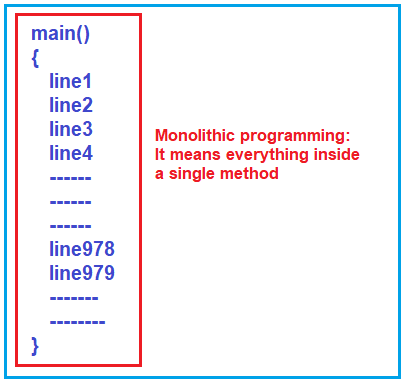So, suppose we break the program into smaller tasks, i.e., into many smaller functions, and each function performs a specific task. In that case, such programming is called Modular Programming or Procedural programming, and this approach is good for development.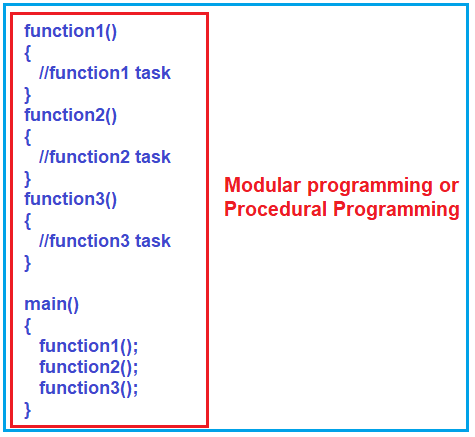As shown in the above image, the first function, i.e., function1(), will perform some specific task, and another function, i.e., function2(), will perform some other task, and similarly, function3() may perform some task. So, in this way, we can break the larger task into smaller, simple tasks, and then we can use them all together inside the main function.

Here, in the Modular Programming Approach, you can break the program into smaller tasks, and you can focus on smaller tasks, finish them, and make them perfect.  This Modular style of programming approach has increased productivity and reusability. For example, if you want the logic of function2 three times inside the main method, then you need to call function2 three times. That means we are reusing the logic defined in function 2. This is called reusability.

##### Modular Programming Examples:

Now, let us focus on modular programming and see how to break a program into functions. Let us write a simple program using a function and learn how to separate the task from the main function.

In the example below, we have implemented the logic to add only two numbers inside the main function.

```#include <stdio.h>
int main ()
{
int x, y;
x = 10;
y = 5;
int z = x + y;
printf ("sum is %d", z);
}
```

As you can see in the above code, first, we declare two variables, x, and y, and then initialize these two variables with values 10 and 5, respectively. Then we add two variables and store the result in another variable, i.e., z and finally print the value of z in the console, which you can see in the output. Let us see how to write the same Program using Function. For a better understanding please have a look at the below image.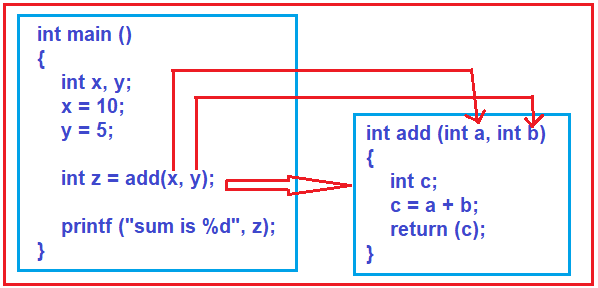As you can see in the above image, we created a function called add, which takes two input parameters, a and b, of type integer. This add function adds the two integer numbers it received as input parameters, stores the result in variable c, and returns that result.

Now, see the main function. From the main function, we are calling the add function. While calling the add function, we are passing two parameters, i.e., x and y (actually, we are passing the values stored in x and y), and these parameters’ values will go into a and b. The add function then adds these two values and returns the result to the calling function (the function is called the add method), i.e., the main method. The main method then stores the result coming from the add method into the variable z and prints the result on the output window. The complete code is given below.

```#include <stdio.h>
int add (int a, int b)
{
int c;
c = a + b;
return (c);
}

int main ()
{
int x, y;
x = 10;
y = 5;
int z = add (x, y);
printf ("sum is %d", z);
}
```
##### Different Parts of a Function:

To understand the different parts of a function, please look at the image below.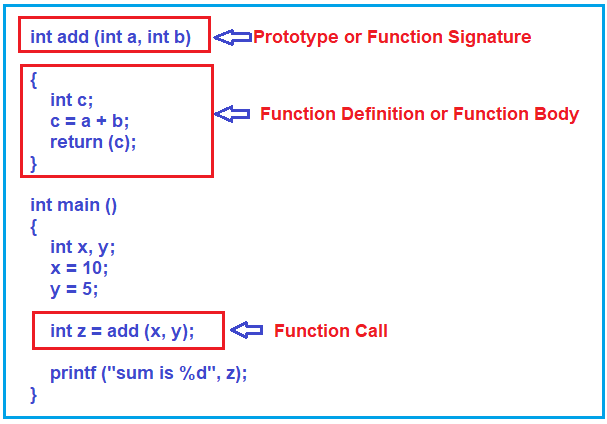##### What are the Parameters of a Function?

Please look at the image below to better understand the function parameters.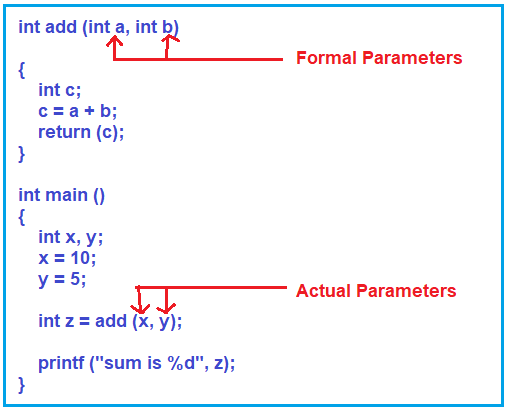As you can see in the above image, we are passing two values, x, and y, to the add function, which takes two parameters (a and b). The parameters (x and y) passing to the add function are called Actual Parameters. The parameters (a and b) that are taken by the add method are called Formal Parameters. When we call the add method, the values of actual parameters are copied to the formal parameters. So, the x value, i.e., 10, is copied to a, and the y value, i.e., 5, is copied to b.

##### How Does it Work Inside the Main Memory?

When the program starts, i.e., when the main method starts its execution, three variables (x, y, and z) are created inside the stack, that is, inside the activation area of the main function. Then, the x and y variables are assigned with values 10 and 15, respectively. Then, the main method is called the add method. Once the add method is called, its own activation area is created inside the stack, and it will have its own variables, i.e., variables a, b, and c are created inside this activation area. Then, the values of x, i.e., 10, and y, i.e., 5, passed to add function are copied to the variables a and b, respectively. Then the add method adds the two numbers and the result is 15, which is stored in the variable c, and that result, i.e., 15, is returned from the add method. The result from the add method is stored in the variable z, which will be printed in the console window. For a better understanding, please have a look at the following image.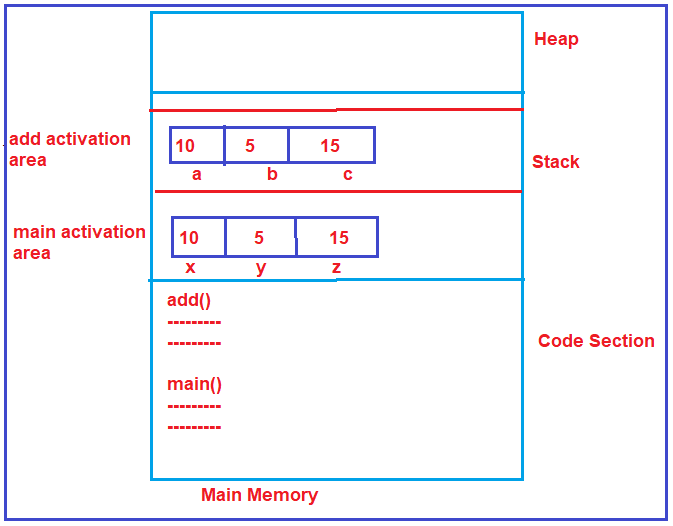Note: One more point you need to remember is that one function cannot access the variables of other functions. I hope you understand the basics of Functions in the C Program. Now, let us proceed and understand the function in detail with more examples.

##### What is a Function in C Language?

A function is a self-content block of 1 or more statements or a subprogram designed for a particular task.  A C Program comprises one or more functions, one of which must be named as the main. The program’s execution always starts and ends with the main function, but we can call other functions to do special tasks from the main function. The following are the key concepts related to function:

Definition: Specifies what the function does. It includes the function name, return type, parameters (if any), and the function’s body (code block).

Declaration: Also known as the prototype, it tells the compiler about the function name, return type, and parameters. It’s needed if the function is defined after the main function or in another file.

Syntax
returnType functionName(parameter1Type parameter1Name, parameter2Type parameter2Name, …) {
// Function body
// …
return value; // if the return type is not void
}

Passing Parameters

• Call by Value: The actual value is passed. Changes made to the parameter inside the function don’t affect the argument.
• Call by Reference: The address of the variable is passed. Changes made to the parameter affect the passed argument.

Return Type: Determines the type of value the function returns. It can be any data type, including void, which means the function doesn’t return anything.

Recursion: Functions can call themselves. This is known as recursion, and it’s often used for tasks like sorting and traversing data structures.

Scope: Where the function is accessible.

Lifetime: How long the function exists in memory. Local variables inside functions are generally auto-storage classes and have a scope limited to the function.

Types of Functions

• Standard Library Functions: Predefined in C (e.g., printf(), scanf(), strcpy()).
• User-Defined Functions: Created by the programmer to perform specific tasks.

Note: The function cannot use the variables of other functions (even the main function). So, if you want the function to use some variable of another function, then you have passed that variable as an argument to the function.

###### Example to understand Functions in C Language:
```#include <stdio.h>

void name();	    /*function declaration */
void message();		/*function declaration */
void main()
{
name();
message();
}

void name()		   /*function definition */
{
printf("Ram ");
}

void message ()	   /*function definition */
{
name();
printf("Hello ");
}```

Output: Ram Ram Hello

In the above piece of code, the name() and message() are the user-defined functions. From the main() function, we called the name() and message() functions. When the functions are called, they execute and perform their respective tasks.

##### Advantages of Functions in C Language

Functions in C language offer several significant advantages that contribute to efficient and effective programming:

###### Modularity
• Structured Approach: Functions break the program into smaller, manageable segments or modules, making it more structured and understandable.
• Focus on Individual Tasks: Each function can be developed independently, focusing on a specific task.
###### Reusability
• Code Reuse: Once a function is written, it can be reused multiple times throughout the program. This avoids redundancy and saves time.
• Sharing and Maintenance: Reusable functions can be shared across different programs, facilitating easier maintenance and updates.
###### Abstraction
• Hiding Details: Functions abstract the implementation details from the user. Users can utilize the function without knowing the inner workings.
• Simplifying Complexity: By abstracting details, functions make complex processes appear simple, aiding in problem-solving.
###### Ease of Debugging and Testing
• Isolate Issues: Since functions encapsulate specific functionalities, isolating and troubleshooting issues is easier.
• Incremental Testing: Individual functions can be tested separately, making debugging more efficient.
###### Better Organization
• Structured Code: Functions help organize the code logically, making it easier to read and understand.
• Easier Maintenance: Well-organized code is easier to modify and update.
###### Efficient Memory Usage
• Avoiding Repetition: By avoiding repetitive code, functions can lead to more efficient memory usage.
• Dynamic Allocation: Functions can dynamically allocate and free memory, managing memory effectively.
###### Scope Management
• Local Variables: Functions allow for local variables, limiting the scope and preventing unwanted side effects on other program parts.
• Parameter Passing: Functions can receive data through parameters, allowing for more flexible and dynamic code.
###### Scalability
• Easier Enhancements: Adding new features to a program is more manageable when using functions, as changes can be made in small, specific areas.
• Adaptability: Functions can be modified or extended to suit new requirements without having to overhaul the entire program.
###### Collaboration
• Teamwork: Different programmers can work on separate functions simultaneously in a team environment, enhancing collaboration and productivity.
###### Facilitates Recursion
• Elegant Solutions: Functions enable recursive solutions, which can be more elegant and efficient for problems like traversing data structures or implementing algorithms.